NEET  >  Power in an AC Circuit

# Power in an AC Circuit - Notes | Study Physics Class 12 - NEET

 1 Crore+ students have signed up on EduRev. Have you?

7. Power in an AC circuit

In case of a steady current the rate of doing work is given by,

P = Vi

In an alternating circuit, current and voltage both vary with time, so the work done by the source in time interval dt is given by

dW= Vidt

Suppose in an ac, the current is leading the voltage by an angle φ. Then we can write,

V = V0 sinωt

and = i0 sin(ωt + φ)

dW = V0i0 sin ωt sin (ωt + φ) dt

= V0 i0 (sin2 ωt cos f + sinωt cos ωt sin φ) dt

The total work done in a complete cycle is

W = V0i0 cos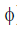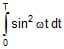+ V0i0sin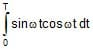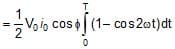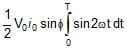=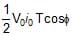The average power delivered by the source is, therefore,

P =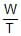=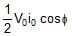=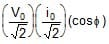= Vrms irms cos φ

or <P>one cycle = Vrms irms cos φ

Here, the term cos φ is known as power factor.

It is said to be leading if current leads voltage, lagging if current lags voltage. Thus, a power factor of 0.5 lagging means current lags the voltage by 60° (as cos-10.5 = 60°). The product of Vrms and irms gives the apparent power. While the true power is obtained by multiplying the apparent power by the power factor cos φ. Thus,

and apparent power = Vrms × irms

True power = apparent power × power factor

For φ= 0°, the current and voltage are in phase. The power is thus, maximum (Vrms× irms). For

φ = 90°, the power is zero. The current is then stated as wattless. Such a case will arise when resistance in the circuit is zero. The circuit is purely inductive or capacitive.

The document Power in an AC Circuit - Notes | Study Physics Class 12 - NEET is a part of the NEET Course Physics Class 12.
All you need of NEET at this link: NEET

## Physics Class 12

157 videos|452 docs|213 tests

## Physics Class 12

157 videos|452 docs|213 tests

### How to Prepare for NEET

Read our guide to prepare for NEET which is created by Toppers & the best Teachers

Track your progress, build streaks, highlight & save important lessons and more!

,

,

,

,

,

,

,

,

,

,

,

,

,

,

,

,

,

,

,

,

,

;